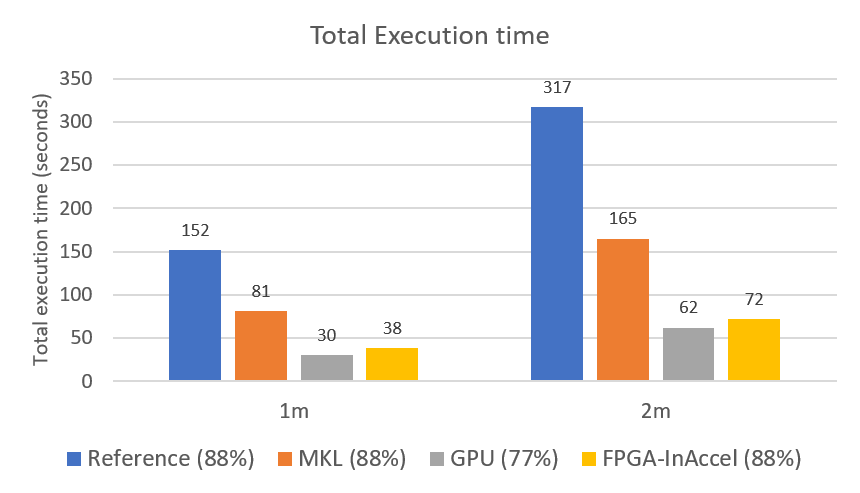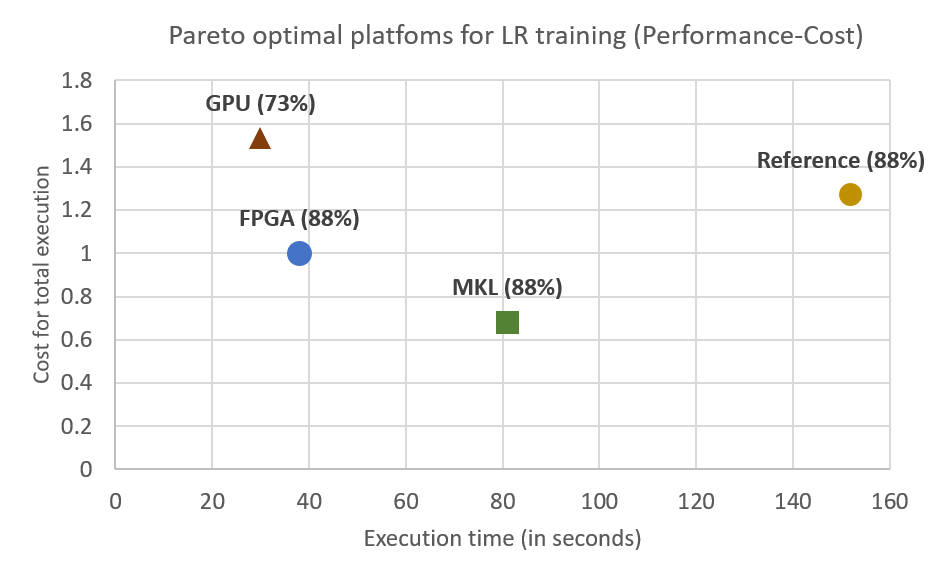#.

A use case on Logistic regression training

Over the last few years there are several efforts for more powerful computing platforms to face the challenges imposed by emerging applications like machine learning. General purpose CPUs have been developed specialized ML modules, GPUs and FPGAs with specialized engines are around the corner. Several startups develop novel ASICs specialized for ML applications and Deep Neural networks.

In this article we perform a comparison of 3 different platforms available on the cloud (general purpose CPUs, GPUs and FPGAs). We evaluate the performance in terms of total execution time, accuracy and cost.

For this benchmark we have selected logistic regression as it is one of the most widely used algorithm for classification. Logistic Regression is used for building predictive models for many complex pattern-matching and classification problems. It is used widely in such diverse areas as bioinformatics, finance and data analytics. Logistic regression measures the relationship between the categorical dependent variable and one or more independent variables by estimating probabilities using a logistic function.

Logistic Regression was chosen because it’s arguably the most popular algorithm for building predictive analytics use cases and its iterative procedure when fitting the model, which allows us to extract better results on this comparison.

In this case we evaluate the training of the MNIST dataset with 10 classes and 1 million dataset.

The purpose of these notebooks is to compare and evaluate the performance of a Logistic Regression model using three implementations, Python’s Sklearn package (alongside Intel’s Math Kernel Library), Rapids cuml library and InAccel Sklearn-like package. The first one is the widely used Data Science library, Intel’s MKL is a cpu math processing accelerated framework, while the others are newer solutions built on top of GPU and FPGA accelerators respectively.

We compared the training in 4 different platforms:

1. Reference: Intel Xeon Skylake SP (r5.2xlarge with original code)
2. MKL: Intel Xeon Skylake SP (r5.2x large using MKL libraries)
3. GPU: NVIDIA® V100 Tensor Core (p3.2x large RAPIDS library)
4. FPGA: FPGA (f1.2x using InAccel ML suite)

In the case of general purpose CPUs we use both the original code (without optimized libraries) and the Intel MKL library for optimization of ML kernels. In the case of GPUs, we use the RAPIDS framework and in the case of FPGAs we use our own ML suite for logistic regression available from InAccel.

The following figure depicts the performance of each platform (total execution time). As you can see in terms of performance, GPU achieves the best performance compared to the rest of platforms. However, the accuracy in this case is only 73% while the rest of the platforms can achieve up to 88% accuracy. So in terms of accuracy, FPGAs using the InAccel ML suite can achieve the optimum performance and very high accuracy.Total execution time for ML training of logistic regression (MNIST). In parenthesis, the accuracy achieved for each platform/algorithm.

However, cost is also very important for enterprises and data scientists. In this case we compare the performance vs cost trade off using these 4 platforms. The cost of each platform is shown below:

• r5.2xlarge: \$0.504/h
• p3.2xlarge: \$3.06/h
• f1.2xlarge: \$1.65/h

In the following figure we show the performance (total execution time) and total cost for the ML training for these 4 platforms.Performance vs Cost for the training of Logistic regression using MNIST (In the parenthesis you can see the accuracy each model achieved)

As you can see in this figure, FPGAs on the cloud (f1.2xlarge on this case with InAccel ML suite) achieves the best combination in terms of performance-accuracy and cost. Optimized libraries for GPP (MKL) achieve the most cost-efficient solution but the performance is not as high as using accelerators. GPUs can achieve better performance but the cost is much higher and in this case the accuracy is not acceptable in many applications.

Views: 1049

Comment

### You need to be a member of Data Science Central to add comments!

Join Data Science Central